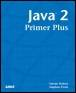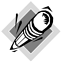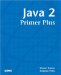# Method OverloadingJava™ 2 Primer PlusBy Steven Haines, Steve Potts Table of Contents Chapter 5.  Methods

Consider defining a method called square that returns the square of two numbers, which has been done repeatedly in this chapter. The examples thus far have worked on ints, but what happens when we want to square a long or a double? Do you create different methods with different names to solve the problem?

` int squareInt( int i )  long squareLong( long l )  double squareDouble( double d ) `

That is perfectly legal, but when you are writing your code, and subsequently reading it back later, do you care whether you are squaring an int or a long? It would be nice to have the method square return the square of all the previous types.

Unfortunately, because of how variables are stored in memory, there is no way to write a single method that handles all these cases, but Java enables you to reuse the same method name. Remember that a method is defined by its signature; the signature includes the method name and its parameter list. As long as methods differ in their signature, the compiler treats them as different methods.

This means that you are free to define methods with the same name as long as their parameter list is different. Here are three square methods that solve this problem:

` int square( int i )  long square( long l )  double square( double d ) `

All three methods are named square, but their parameter lists are different: the first is a single int, the second is a long, and the last is a double. The rules are satisfied and the compiler will treat these three methods differently. The process of defining multiple methods with the same name but differing signatures is referred to as method overloading. Listing 5.5 shows a complete example of these three methods.

##### Listing 5.5 SquareOverload.java
` public class SquareOverload {    public static int square( int n ) {      System.out.println( "Integer Square" );      return n*n;    }    public static long square( long l ) {      System.out.println( "Long Square" );      return l*l;    }    public static double square( double d ) {      System.out.println( "Double Square" );      return d*d;    }    public static void main( String[] args ) {      int n = 5;      long l = 100;      double d = 1000.0;      System.out.println( "n squared=" + square( n ) );      System.out.println( "l squared=" + square( l ) );      System.out.println( "d squared=" + square( d ) );    }  } `

When the compiler sees the three different versions of square in Listing 5.5, it treats them as separate methods.

NoteA final note about method signatures is that although the return value is part of the signature, it cannot be the differentiating factor that results in overloaded methods. The following two methods cannot be defined in the same Java class: ` public int square( long l ) { ... } public long square( long l ) { ... } `The compiler only looks at the method name and parameter list, and does not consider the return type.

 TopJava 2 Primer Plus
ISBN: 0672324156
EAN: 2147483647
Year: 2001
Pages: 332

Similar book on Amazon

flylib.com © 2008-2017.
If you may any questions please contact us: flylib@qtcs.net